# Old Flow Plug in page

## Flows plugin

An extension of the Flow Plugin is in the development. It will provide step-wise flow transformation rules.

Event-B, being an event systems formalism does not have a mechanism for explicitly defining event ordering. Although event guards may express any desired event ordering, the ability to have a summary of possible event flows in a concise and compact form is useful for many tasks, for example, code generation and connecting with other formalisms. The flows plugin addresses one aspect of event ordering: it allows a modeller to specify and prove that a given sequence of events does not contradict a given machine specification. More precisely, if we were to execute a machine step-by-step following our prescribed sequence of events we would not discover divergencies and deadlocks not already present in the original machine. In other words, the constraining of event ordering must be such that the overall specification is an Event-B refinement of the original model. Importantly, this means that all the desired model properties proved before are preserved.

Sequential composition of events may be expressed in a number of ways:

• event immediately follows another event; no other events may take place between the composed events.
• event eventually follows an event; thus, although there is an interference from other events, it is guaranteed that the second is eventually enabled.
• event may follow an event; this is the weakest form of connection when we only say that it may be the case that the second event follows the first event; it may happen, however, that some other event interferes and the second event is delayed or is even not enabled ever.

### The Flows Approach

Although the last case may seem the least appealing, it is the one that forms the basis of the flows approach. The primary reason for offering such a weak guarantee is proof effort required for stronger types of connectives. Let us see what it means to provide a formal proof for the various cases of sequential event composition.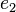$e_2$ "immediately after"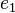$e_1$ requires, among others, the demonstration of a condition that no event other than$e_2$ is enabled in the after-state of$e_1$. This leads to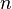$n$ proof obligations where$n$ is the number of machine events. This number of proof obligations is comparable to the effort of demonstrating machine consistency. For a flow expression mentioning "immediately after"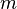$m$ times there would be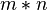$m*n$ proof obligations. Even if only a small fraction of these result in interactive proofs, it would still be impractical for any realistic model.

For the second case, where$e_2$ "eventually follows"$e_1$, one has to prove that an overall effect of any possible interference between the occurrence of$e_1$ and$e_2$ is such that the resultant state is a sub-state of states where$e_2$ is enabled. Seeing all other events as relations on machine state and assuming they are already proved convergent, the effect of event interference is represented by a transitive closure of a disjunction of all interference relations. The result is a rather expensive proof obligation that, almost certainly, would require a manual proof.

Contrastingly, the last case -$e_2$ "may follow"$e_1$ - requires one simple proof obligation that, in most cases, would be discharged without a user assistance: the after-state of$e_1$ should imply the guard of$e_2$. We denote this condition as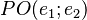$PO(e_1;e_2)$. This style of sequential composition used in the flows method and the following shortcut notation is offered: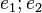$e_1; e_2$ (note the events order).

One generalisation of$e_1;e_2$ is having a vector of events on the right-hand side: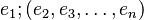$e_1;(e_2, e_3, \dots, e_n)$. It is easy to see how to scale up the proof obligation for this case. Then,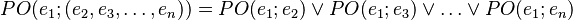$PO(e_1;(e_2, e_3, \dots, e_n)) = PO(e_1;e_2) \lor PO(e_1;e_3) \lor \dots \lor PO(e_1;e_n)$.

The meaning of this extended construct is quite straightforward: there is a choice of possible continuation events after some current event$e_1$. Further, we use the following notation for$e_1;(e_2, e_3, \dots, e_n)$: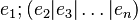$e_1;(e_2 | e_3 | \dots | e_n)$ where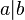$a | b$ reads as "a choice of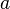$a$ and$b$". Note that expression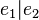$e_1 | e_2$ is not well-formed on its own. Choice of events has a meaning only in a combination with operator$;$.

A flow expression is a list of statements about event ordering.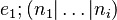$e_1;(n_1 | \dots | n_i)$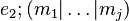$e_2;(m_1 | \dots | m_j)$$\dots$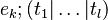$e_k;(t_1 | \dots | t_l)$

The semantics of each such statement is already given above: it is a theorem expressing requirements to the events mentioned in a statement. More precisely, it is an additional proof obligation for machine for which the flow statements are defined. Note that there is no obligation to mention all the machine events in a flow expression.

How expressive is the language of flows? There is, effectively, just one construct but it already covers a form of sequential composition, choice and loop. A loop is expressed by adding a statement to pass control back the beginning of the loop body: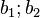$b_1;b_2$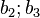$b_2;b_3$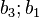$b_3;b_1$

The above is a loop made of a sequential composition of three events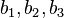$b_1, b_2, b_3$.

#### Concurrency

One important notion missing in the flows language is event concurrency. And here again, the concept of passing control from one event to another (operator$;$) plays the central role. First, we note that concurrency is linked to the idea of a thread of a control. In other words, to define$m$ events as executing concurrently there must be at least$m$ threads of control. Another observation is that there should be a way to start (or unblock) and terminate (block) a thread. In this case, by starting a thread we understanding passing a control to a continuation that has one additional thread of control (an alternative is having all threads started by an initialisation and then being unblocked and blocked when necessary). Correspondingly, termination of thread is a continuation that has one less thread of control. Terminating the last thread is the termination of the whole system.

To construct a new thread, some current tread is split into two threads. In Event-B terms, this corresponds to passing the control from an event to a pair of events such that they may execute in any order, that is, hey are both enabled and do not assign to the same model variables. We call such events independent. Let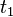$t_1$ and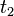$t_2$ be some distinct independent events. Then notation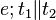$e;t_1 \| t_2$ states that after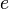$e$ both$t_1$ and$t_2$ are enabled.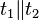$t_1 \| t_2$ reads as "$t_1$ concurrently with$t_2$". The semantics of this construct is quite simple: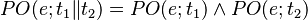$PO(e;t_1 \| t_2) = PO(e;t_1) \land PO(e;t_2)$.

Thread termination is a symmetric case of thread creation. Concurrent events appear on the left-hand side of a sequential composition with an event: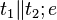$t_1 \| t_2; e$. The meaning of this construct is that once$t_1$ and$t_2$ stopped,$e$ is enabled. Since$t_1$ and$t_2$ are independent, it is enough to separately demonstrate that$t_1$ enables$e$ and$t_2$ enables$e$: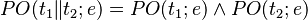$PO(t_1 \| t_2;e) = PO(t_1;e) \land PO(t_2;e)$.

The way we have defined thread construction does not make it clear how to define a thread that starts with a choice of events, for example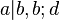$a | b, b;d$. This limitation, however, is easy to overcome by noticing that the skip event may be used to reduce the case of a thread starting with a choice to thread that has a single initial event: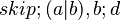$skip;(a | b), b;d$. Since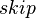$skip$ does no alter state, the overall behaviour is the same. This way, we are able to treat a general case: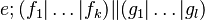$e;(f_1 | \dots | f_k) \| (g_1 | \dots | g_l)$

The semantics of the construct is defined as follows: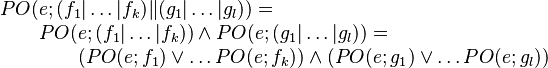$\begin{array}{l} PO(e;(f_1 | \dots | f_k) \| (g_1 | \dots | g_l)) = \\ \qquad PO(e;(f_1 | \dots | f_k)) \land PO(e;(g_1 | \dots | g_l)) = \\ \qquad \qquad (PO(e;f_1) \lor \dots PO(e;f_k)) \land (PO(e;g_1) \lor \dots PO(e;g_l)) \end{array}$

The same thinking is applied to thread termination case. For a more readable notation, the sequential composition operation may be generalised to an associative version: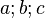$a;b;c$

The above simply stands for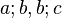$a;b, b;c$. It is also easy to see how to scale this up to for the case of choice construct. However, loop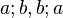$a;b, b;a$ would be defined as - using a new operator * -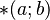$*(a;b)$ rather than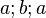$a;b;a$. The is due to the fact that we want to be able to make a distinction between an event of a model and an event of a flow expression. Such a generalisation makes it possible to include the same event more than once in a flow expression and define event parameters in flows.

To account for event parameter, the basic PO rule$PO(e_1;e_2)$ is now extended to accept two parameters: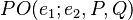$PO(e_1;e_2, P, Q)$. Here,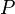$P$ and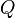$Q$ are predicates defined on model variables and also on the parameters of their respective events.$P$ and$Q$ are, essentially, additional event guards and that is also how they appear in a proof obligation.

#### Event Compositionality PO

Finally, we give a formal definition of$PO(e_1;e_2, P, Q)$. Remember, that$e_1;e_2$ was defined as an event may follow another event. A way to formally demonstrate this is to show that an after-state of the first event always enables the guard of the next event.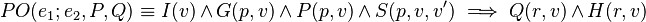$PO(e_1;e_2, P, Q) \equiv I(v) \land G(p, v) \land P(p, v) \land S(p, v, v') \implies Q(r, v) \land H(r, v)$

where$p$ and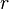$r$ are the parameters of the first and the second event, correspondingly.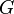$G$ and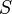$S$ are the guard and before-after predicate of the first event whereas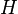$H$ is the guard of the second event.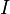$I$ is the model invariant and$P$ and$Q$ are the parameter constraining predicates from a flow expression.

#### Update site

http://iliasov.org/flowplugin/1.2/